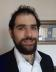## Pizza Party for 100

Hi all, this is my first blog post! Yay!

Now that that's out of the way, I'd just like to go over my solution for this week's Perl weekly challenge.

The first challenge was to divide a pie between 100 people, in a manner where the first guest gets 1/100 (i.e. 1%) of the pie, and the second gets 2/100 (i.e. 2%) of what remained, etc etc. The question is, who got the biggest piece of pie.

If I were better at math, I would prove that the answer is the square root of the amount of guests, because that does seem to be the case. Since I'm not, I solved it procedurally, as follows.

We set up our guests. We allow for the user to pass in a value on the command line, to check values other than 100, but default to 100:

``````use v5.22; #that's my perl
my \$guests = shift // 100;
``````

Then, we define a function to slice a piece of the pie:

``````sub cut_a_slice_for {
state \$pie = 1;
my \$slice = (shift() / \$guests ) * \$pie;
\$pie -= \$slice;
return \$slice
}
``````

Here we use `state` to declare a persistent local variable, keeping track of the size of the pie. It then cuts the slice for this guests piece. It's then called in sequence:

``````my \$biggest = {};
for my \$guest ( 1 .. \$guests ) {
my \$piece = cut_a_slice_for(\$guest);
\$biggest = { name => \$guest, size => \$piece } if \$piece > \$biggest->{size}
}
``````

We use an anonymous hash to store the place and the size of the biggest piece seen so far, and then

``````say \$biggest->{name}
``````

print the answer at the end.

> Hi all, this is my first blog post! Yay!

Congratulations. And please write many more if you can. :-)

Congratulation on your first blog. You made me re-work the Cake task in my head when you mentioned the answer is the square root of the amount of guests. I wonder how did you come to that conclusion? I am not saying it is wrong, just curious to know how?

I think that this hypothesis from Veesh is probably right (you need to change the number of guests and the share, so that the last guest gets 100% of the rest), except possibly for the rounding which might offset the result by 1. Trying my code with various values confirms the idea.

With an initial share of 0.001 and 1,000 guests, I get:
Lucky guest: 32
Largest share: 0.0193839288860447
32 is more or less the square root of 1,000.

With 50 guests and an initial share of 0.2:
Lucky guest: 7
Largest share: 0.0902123937792

With 20 guests and an initial share of 0.5:
Lucky guest: 4
Largest share: 0.14535

I've tried to do the math and, if I got it right, it turns out that it is not exactly the square root, but it is pretty close (and the square root is probably right in practical terms).

When guest n comes in, the rest of the cake can be called r. So, guest n gets r * n/100. And what is left after guest n has been served is r - (r * n/100) = r * (100 - n) / 100.

Guest n+1 gets (r * (100 - n) / 100) * (n+1)/100. So guest n+1 gets more than guest n if r * (100 - n) / 100) * (n+1)/100 > r * n/100. Since r is a strictly positive number, we can remove r from both sides and simplify to (100 - n) / 100) * (n+1)/100 > n/100. This leads to: 100 - n² - n > 0. Or: n² + n = 10. So guest n+1 will get more than guest n for n

To find exactly where the function starts to decrease, we can solve the equation: -x² - x + 100 = 0. The discriminant is: delta = 1 + 400 = 401. The roots of the equation are: (1 +/- sqrt(delta) / -2. Disregarding the negative root, we get: x = -0.5 + sqtr(401)/2 = 0.5 + (sqrt(401)/2 = appprox. 10.5. Note that if we use other values (such as 50 guests and 2% shares), the equation will be the same except for the last term which will be 50 instead of 100. And the solution will be essentially the same, except that delta will be 201. This can thus be generalized to any number of guests.

Anyway, the point is that the math says (if I did not goof something in the computation) is that the discriminating factor is not exactly the square root of the number of guests, but half the square root of four times the number of guests + 1, which is pretty damn close, so that the approximation square root of the number of guests is probably good enough in practical terms for any number of guests larger than 2.

Oops, the end of the third paragraph of my previous reply got somehow cut.

... This leads to: 100 - n² - n > 0. Or: n² + n

(Again a problem).

Oops, the end of the third paragraph of my previous reply got somehow cut.

... This leads to: 100 - n² - n > 0.

Or: n² + n less than 100.

We can easily see that guest n+1 will get more than guest n for n equal 3 to 9. In particular, for n = 9, guest 10 will get a larger share than guest 9. Subsequent guests will get less than their immediate predecessor and less than guest 10.I do full-stack development with Mojolicious and Vue.